# High School Physics : Capacitors

## Example Questions

← Previous 1

### Example Question #1 : Capacitors

Which of these is the correct SI unit for capacitance?

Ohms

Newtons

Volts

Explanation:

The correct unit for capacitance is the Farad, named after the English physicist Michael Faraday.

Newtons are used to measure force. Ohms measure resistance and volts measure electrical energy or potential. Joules are a unit of energy, but Farad-Joules are not a recognizable unit.

### Example Question #2 : Capacitors

By using a dielectric, it is possible to store more energy in a capacitor. Why is this?

The dielectric replaces air, which breaks down when the electric fields get strong enough

The dielectric can store excess energy

The dielectric provides a direct energy source to the capacitor

The dielectric changes the distance between the two plates of the capacitor

The dielectric replaces air, which breaks down when the electric fields get strong enough

Explanation:

Dielectrics are insulating materials that can be polarized, and can be used to increase the separability of the two plates of a capacitor. When a dielectric is absent, air is present between the capacitor plates. At high voltages, when the electric field between the plates is incredibly strong, air begins to break down and becomes unable to prevent the flow of electricity between the capacitor plates. When this occurs, the capacitor cannot store energy, nor can it properly function. The presence of a dielectric eliminates this problem, allowing the capacitor to store much more energy.

### Example Question #1 : Calculating Total Capacitance

Three capacitors are in series. They have capacitances of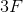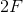, and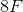, respectively. What is their total capacitance?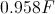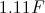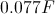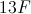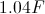Explanation:

For capacitors in series the formula for total capacitance is: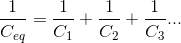Note that this formula is similar to the formula for total resistance in parallel. Using the values for each individual capacitor, we can solve for the total capacitance.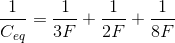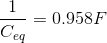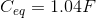### Example Question #41 : Electricity And Magnetism

Three capacitors are in parallel. They have capacitance values of, and. What is their total capacitance?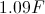Explanation:

For capacitors in parallel the formula for total capacitance is: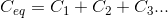Note that this formula is similar to the formula for total resistance in series. Using the values for each individual capacitor, we can solve for the total capacitance.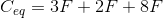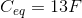### Example Question #1 : Calculating Total Capacitance

Three capacitors, each with a capacity of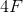are arranged in parallel. What is the total capacitance of this circuit?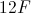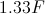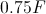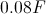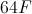Explanation:

The formula for capacitors in parallel is:Our three capacitors all have equal capacitance values. We can simply add them together to find the total capacitance.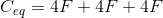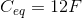### Example Question #4 : Calculating Total Capacitance

What is the total capacitance of a series circuit with capacitors of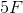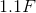, and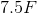?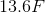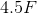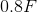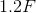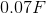Explanation:

The total capacitance of a series circuit isPlug in our given values.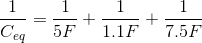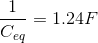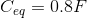### Example Question #5 : Calculating Total Capacitance

Calculate the total capacitance of a circuit with the following three capacitors in parallel.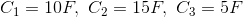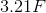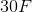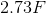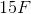Explanation:

To calculate the total capacitance for capacitors in parallel, simply sum the value of each individual capacitor.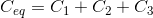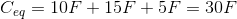### Example Question #6 : Calculating Total Capacitance

Calculate the total capacitance of a circuit with the following three capacitors in series.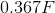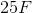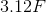Explanation:

To find the total capacitance for capacitors in series, we must sum the inverse of each individual capacitance and take the reciprocal of the result.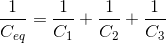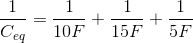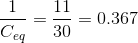Remember, you must still take the final reciprocal!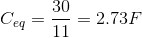### Example Question #1 : Using Capacitor Equations

Three capacitors in parallel have a capacity of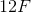, and, respectively. What is the total capacitance?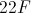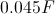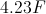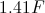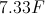Explanation:

The formula for capacitors in parallel is:Plug in our given values: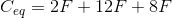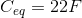### Example Question #1 : Using Capacitor Equations

Three capacitors in series have a capacity of, and, respectively. What is the total capacitance?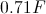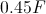Explanation:

The formula for capacitors in series is:Plug in our given values: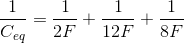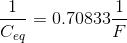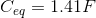← Previous 1